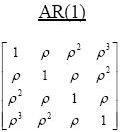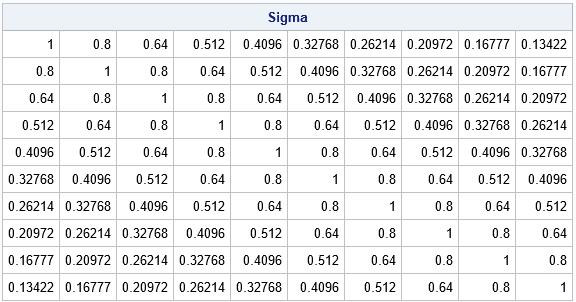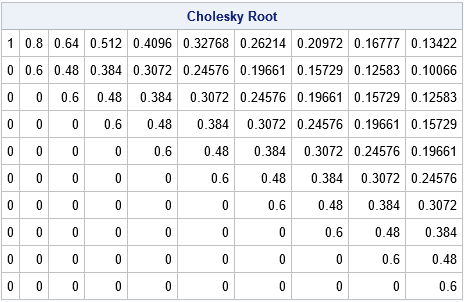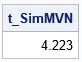It is sometimes necessary for researchers to simulate data with thousands of variables. It is easy to simulate thousands of uncorrelated variables, but more difficult to simulate thousands of correlated variables. For that, you can generate a correlation matrix that has special properties, such as a Toeplitz matrix or a first-order autoregressive (AR(1)) correlation matrix. I have previously written about how to generate a large Toeplitz matrix in SAS. This article describes three useful results for an AR(1) correlation matrix:

• How to generate an AR(1) correlation matrix in the SAS/IML language
• How to use a formula to compute the explicit Cholesky root of an AR(1) correlation matrix.
• How to efficiently simulate multivariate normal variables with AR(1) correlation.

### Generate an AR(1) correlation matrix in SASThe AR(1) correlation structure is used in statistics to model observations that have correlated errors. (For example, see the documentation of PROC MIXED in SAS.) If Σ is AR(1) correlation matrix, then its elements are constant along diagonals. The (i,j)th element of an AR(1) correlation matrix has the form Σ[i,j] = ρ|i – j|, where ρ is a constant that determines the geometric rate at which correlations decay between successive time intervals. The exponent for each term is the L1 distance between the row number and the column number. As I have shown in a previous article, you can use the DISTANCE function in SAS/IML 14.3 to quickly evaluate functions that depend on the distance between two sets of points. Consequently, the following SAS/IML function computes the correlation matrix for a p x p AR(1) matrix:

```proc iml; /* return p x p matrix whose (i,j)th element is rho^|i - j| */ start AR1Corr(rho, p); return rho##distance(T(1:p), T(1:p), "L1"); finish;   /* test on 10 x 10 matrix with rho = 0.8 */ rho = 0.8; p = 10; Sigma = AR1Corr(rho, p); print Sigma[format=Best7.];```### A formula for the Cholesky root of an AR(1) correlation matrix

Every covariance matrix has a Cholesky decomposition, which represents the matrix as the crossproduct of a triangular matrix with itself: Σ = RTR, where R is upper triangular. In SAS/IML, you can use the ROOT function to compute the Cholesky root of an arbitrary positive definite matrix. However, the AR(1) correlation matrix has an explicit formula for the Cholesky root in terms of ρ. This explicit formula does not appear to be well known by statisticians, but it is a special case of a general formula developed by V. Madar (Section 5.1, 2016), who presented a poster at a Southeast SAS Users Group (SESUG) meeting a few years ago. An explicit formula means that you can compute the Cholesky root matrix, R, in a direct and efficient manner, as follows:

```/* direct computation of Cholesky root for an AR(1) matrix */ start AR1Root(rho, p); R = j(p,p,0); /* allocate p x p matrix */ R[1,] = rho##(0:p-1); /* formula for 1st row */ c = sqrt(1 - rho**2); /* scaling factor: c^2 + rho^2 = 1 */ R2 = c * R[1,]; /* formula for 2nd row */ do j = 2 to p; /* shift elements in 2nd row for remaining rows */ R[j, j:p] = R2[,1:p-j+1]; end; return R; finish;   R = AR1Root(rho, p); /* compare to R = root(Sigma), which requires forming Sigma */ print R[L="Cholesky Root" format=Best7.];```You can compute an AR(1) covariance matrix from the correlation matrix by multiplying the correlation matrix by a positive scalar, σ2.

### Efficient simulation of multivariate normal variables with AR(1) correlation

An efficient way to simulate data from a multivariate normal population with covariance Σ is to use the Cholesky decomposition to induce correlation among a set of uncorrelated normal variates. This is the technique used by the RandNormal function in SAS/IML software. Internally, the RandNormal function calls the ROOT function, which can compute the Cholesky root of an arbitrary positive definite matrix.

When there are thousands of variables, the Cholesky decomposition might take a second or more. If you call the RandNormal function thousands of times during a simulation study, you pay that one-second penalty during each call. For the AR(1) covariance structure, you can use the explicit formula for the Cholesky root to save a considerable amount of time. You also do not need to explicitly form the p x p matrix, Σ, which saves RAM. The following SAS/IML function is an efficient way to simulate N observations from a p-dimensional multivariate normal distribution that has an AR(1) correlation structure with parameter ρ:

```/* simulate multivariate normal data from a population with AR(1) correlation */ start RandNormalAR1( N, Mean, rho ); mMean = rowvec(Mean); p = ncol(mMean); U = AR1Root(rho, p); /* use explicit formula instead of ROOT(Sigma) */ Z = j(N,p); call randgen(Z,'NORMAL'); return (mMean + Z*U); finish;   call randseed(12345); p = 1000; /* big matrix */ mean = j(1, p, 0); /* mean of MVN distribution */   /* simulate data from MVN distribs with different values of rho */ v = do(0.01, 0.99, 0.01); /* choose rho from list 0.01, 0.02, ..., 0.99 */ t0 = time(); /* time it! */ do i = 1 to ncol(v); rho = v[i]; X = randnormalAR1(500, mean, rho); /* simulate 500 obs from MVN with p vars */ end; t_SimMVN = time() - t0; /* total time to simulate all data */ print t_SimMVN;```The previous loop generates a sample that contains N=500 observations and p=1000 variables. Each sample is from a multivariate normal distribution that has an AR(1) correlation, but each sample is generated for a different value of ρ, where ρ = 0.01. 0.02, ..., 0.99. On my desktop computer, this simulation of 100 correlated samples takes about 4 seconds. This is about 25% of the time for the same simulation that explicitly forms the AR(1) correlation matrix and calls RandNormal.

In summary, the AR(1) correlation matrix is an easy way to generate a symmetric positive definite matrix. You can use the DISTANCE function in SAS/IML 14.3 to create such a matrix, but for some applications you might only require the Cholesky root of the matrix. The AR(1) correlation matrix has an explicit Cholesky root that you can use to speed up simulation studies such as generating samples from a multivariate normal distribution that has an AR(1) correlation.

ShareDistinguished Researcher in Computational Statistics

Rick Wicklin, PhD, is a distinguished researcher in computational statistics at SAS and is a principal developer of SAS/IML software. His areas of expertise include computational statistics, simulation, statistical graphics, and modern methods in statistical data analysis. Rick is author of the books Statistical Programming with SAS/IML Software and Simulating Data with SAS.

1.Thank you for an informative article. Do you have any suggestion for simulating multivariate normal data with an AR(p) correlation structure?

•It sounds like you are asking how to simulate a multivariate AR(p) series. You can use the VARMASIM function in SAS/IML, which simulates a VARMA(p, q) series.

•I get the error message below from the execution of the following statement:
l=t(root(cor));
ERROR: The matrix operation is not supported for matrices of size greater than 2147483647 bytes.
"cor" is 40,000x40,000 correlation matrix. It is not an AR(1) correlation matrix.
I have set the memsize to 24G. I am using SAS/IML 14.3 in the X64_10PRO WIN 10 platform.
Any help with this issues is appreciated.
Thank you.

•1. I don't think you can factor a matrix this large in SAS/IML 14.3. The version of PROC IML in SAS IML in Viya 3.5 (or later) supports larger matrices.
2. A Cholesky factorization of a matrix this size will take a long time, even if you use the multithreaded algorithm in Viya. For example, just now I computed the factorization of a 20,000 x 20,000 matrix and it took 2.5 minutes on my PC.
3. Each 40,000 x 40,000 matrix requires 11.9 GB of RAM. (Round up to 12 GB). Therefore, you would need at least 36 GB to hold COR, the temporary Cholesky root, and the transpose. And then, presumably, you want to actually DO something with the matrix, which would require additional large matrices or vectors.

•Thank you Rick for the quick answer. That is helpful. I am trying to figure out my options for the next steps. So, can you confirm that SAS IML in Viya 3.5 (or later) would be able to handle such a matrix (with the appropriate GBs or RAM)? Is there another alternative to the root function? The final goal is to simulate multivariate normal random variables.
Thanks again,
Ardian

•Yes, I used the ROOT function to factor a 40,000 x 40,000 matrix (on SAS IML in Viya 4.0), but it took about 20 minutes.

Before you worry about whether you can factor a large matrix, you should think about the total time required by your simulation study. Presumably, the 40,000 MVN variables are going to be used in some analysis that will be repeated many times. You should time the analysis for smaller number of variables to estimate the time that will be required for 40,000 variables. For example, run your COMPLETE simulation study (all iterations) for 2500, 5000, 7500, and 10000 variables. Fit a cubic polynomial to the Time vs Size graph to predict how long the study will take for 40,000 variables. If the predicted time is huge, you will have to revise your expectations about how large of a system you can simulate. Good luck.